# Calculus 1 : Meaning of Functions

## Example Questions

### Example Question #61 : How To Find The Meaning Of Functions

Find the slope of the tangent line equation for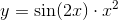when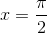.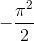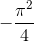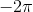Explanation:

To find the slope, we need to differentiate the given function. By product rule and chain rule, we have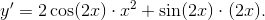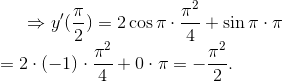### Example Question #61 : How To Find The Meaning Of Functions

Function

What is a function?

A function is an equation with at least two unknowns such as x and y

A function is a relationship that can produce multiple output values for each single input value

A function is a matematical equation with one or, more variables

None of the above

A function is a relationship that assigns to each input value a single output value

A function is a relationship that assigns to each input value a single output value

Explanation:

You could think of a function as a machine that takes in some number, performs an operation on it, and then spits out another number

### Example Question #63 : Meaning Of Functions

Identify Function

Which one of the following is not a function?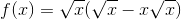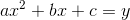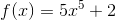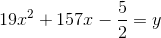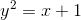Explanation:

For any value ofin, there will be two values of.  So it is not a function.

### Example Question #62 : How To Find The Meaning Of Functions

Type of Functions

What type of function is this: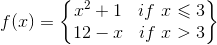Linear

Rational

Polynomial

Piecewise

Piecewise

Explanation:

Piecewise functions are a special type function in which the formula changes for differentvalues.

### Example Question #2814 : Calculus

Find the domain of the function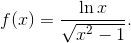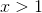or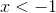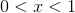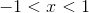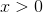orExplanation: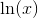is defined when, and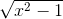is defined when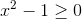. Since the radical part is in the denominator, it cannot be. Therefore, we need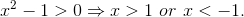Combining this domain with, we get### Example Question #66 : Meaning Of Functions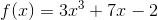What is the slope of this curve at?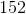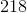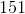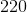Explanation:

To find the slope of a curve at a certain point, you must first find the derivative of that curve.

The derivative of this curve is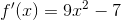.

Then, plug inforinto the derivative and you will getas the slope ofat.

### Example Question #64 : How To Find The Meaning Of Functions

What are the critical points of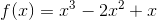?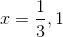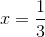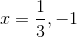Explanation:

To find the critical points of a function, you need to first find the derivative and then set that equal to 0. To find the derivative, multiply the exponent by the leading coefficient and then subtract 1 from the exponent. Therefore, the derivative is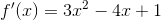. Set that equal to 0 and then factor so that you get: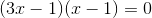. Solve for x in both expressions so that your answer is:.

### Example Question #63 : How To Find The Meaning Of Functions

Finding when the second derivative is positive tells us what about a function?

When the function is decreasing.

When the function is increasing.

When the function is concave down.

When the function is concave up.

When the function is concave up.

Explanation:

The first derivative of a function tells us about the slope and the second derivative tells us about concavity. Thus, when the second derivative is positive, it tell us that the concavity is upward. Therefore, a postive second derivative means the function is concave up.

### Example Question #64 : How To Find The Meaning Of Functions

Given the position function below, what does evaluating it attell us?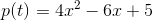It tells us that at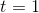, the position of our object is.

It cannot be determined.

It tells us that at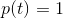, the time our object has been moving is.

It tells us that at, the slope of our function is.

It tells us that at, the position of our object is.

Explanation:

When asked to evaluate the function, you must realize that you are going to be plugging in a value for t. Therefore, we need to figure out what the output value is telling us when t=1. Since we are simply given the position function, adn now asked to integrate it or differentiate it, our output will simply tell us the position of our object when t=1. Thus,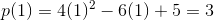### Example Question #2822 : Calculus

Evaluate the following limit: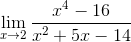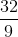Does not exist.Explanation:

When evaluating the limit through direct substitution, one ends with the indeterimate form 0/0, therefore another approach must be followed. Two approaches would work here:

1.

One could use L'Hospital's Rule, which states that if a function is indeterminate when evaluating, one should then take the derivative of the top function over the derivative of the bottom.

In this case one would find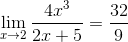2.

Alternatively, one could simply factor both the numerator and the denomenator, removing common factors.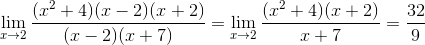### All Calculus 1 Resources